# Electronics and Communication Engineering - Electromagnetic Field Theory

26.
If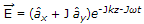and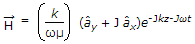the time average Poynting vector is
null vector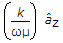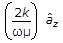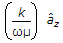Explanation: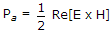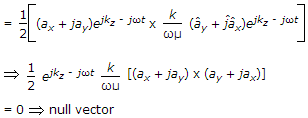27.
Calculate the wave number in waveguide having dimensions 4 x 2 cm at 3 GHz
6.28
62.8
8
0
Explanation: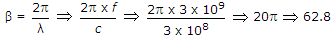.

28.
For a transmission line with homogeneous dielectric the capacitance per unit length is 'c', the relative permittivity of the dielectric is 'εr' and velocity of light in free space is 'v' the characteristic impedance z0 is equal to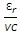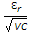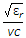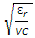Explanation: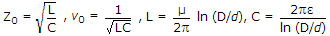.

29.
The I/P impedance of a short circuited quarter wave long transmission line is
purely reactive
purely resistive
depend on the characteristic impedance of the line
none of the above
Explanation:

Because for irrotational, ∇ x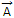= 0.

30.
A dipole antenna of λ/8 length has an equivalent total loss resistance of 1.5 Ω. The efficiency of the antenna is
0.89159%
8.9159%
89.159%
891.59%
Rr = 80p2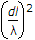, Re = 1.5 Ω,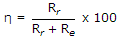.